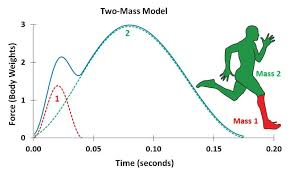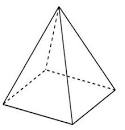## How to Calculate and Solve for Force | MotionThe image above represents force.

To compute for force, two essential parameters are needed and these parameters are mass (m) and acceleration (a).

The formula for calculating force:

F = ma

Where;

F = Force
m = Mass
a = Acceleration

Let’s solve an example;
Find the force when the mass is 12 and the acceleration is 26?

This implies that;

m = Mass = 12
a = Acceleration = 26

F = ma
F = 12 x 26
F = 312

Therefore, the force is 312 N.

Calculating for Mass when the Force and the Acceleration is Given.

m = F / a

Where;

m = Mass
F = Force
a = Acceleration

Let’s solve an example;
Find the mass when the force is 100 and the acceleration is 20.

This implies that;

F = Force = 100
a = Acceleration = 20

m = F / a
m = 100 / 20
m = 5

Therefore, the mass is 5.

## How to Calculate and Solve for the Height, Base Edge and Area of a Square Pyramid | Nickzom CalculatorThe image above is a square pyramid.

To compute the area of a square pyramid, two essential parameters are needed and this parameters are base edge (a) and height (h).

The formula for calculating the area of a square pyramid:

A = a² + 2a√(( / 4) + h²)

Where;
A = Area of a square pyramid
a = Base edge of the square pyramid
h = Height of the square pyramid

Let’s solve for an example;
Find the area of a square pyramid when the base edge (a) given is 8 cm with a height (h) of 12 cm.

This implies that;
a = Base edge of the square pyramid = 8 cm
h = Height of the square pyramid = 12 cm

A = a² + 2a√(( / 4) + h²)
A = 8² + 2 x 8√(( / 4) + 12²)
A = 64 + 16√((64 / 4) + 144)
A = 64 + 16√(16 + 144)
A = 64 + (16)(√160)
A = 64 + (16)(12.65)
A = 64 + 202.3857
A = 266.386

Therefore, the area of the square pyramid is 266.386 cm2.